# Word2vec数学原理全家桶

Mikolov的两篇文章中涉及word2vec的细节甚少. 有不少人都对此模型作出了更加详细的解释, 本文主要沿着Rong, X.word2vec Parameter Learning Explained这篇文章的思路来整理一下，很多公式参考于这篇文章。

• Mikolov, T.(2013). Distributed Representations of Words and Phrases and their Compositionality.
• Mikolov, T.(2013). Efficient Estimation of Word Representations in Vector Space.
• Rong, X. (2014). word2vec Parameter Learning Explained.

## 背景

### 神经网络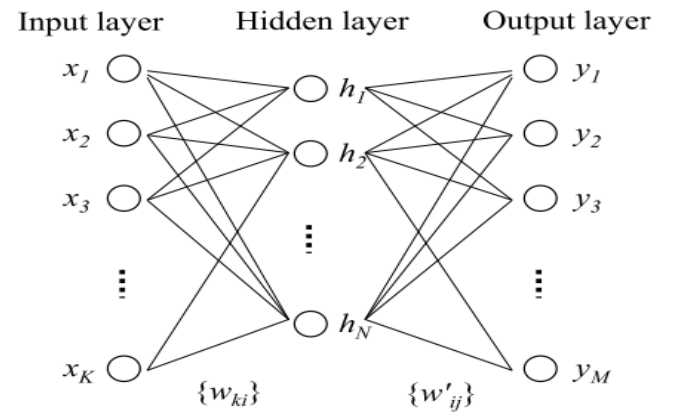### 神经语言模型

$P(sen=(w_1, w_2, .., w_m)) = P(w_1)P(w_2|w_1)P(w_3|w_2,w_1)...P(w_m|w_{m-1}..w_1)$

$P(w_t|w_1,w_2,..,w_{t-1})=P(w_t|w_{t-1}, w_{t-2}...w_{t-(n+1)})$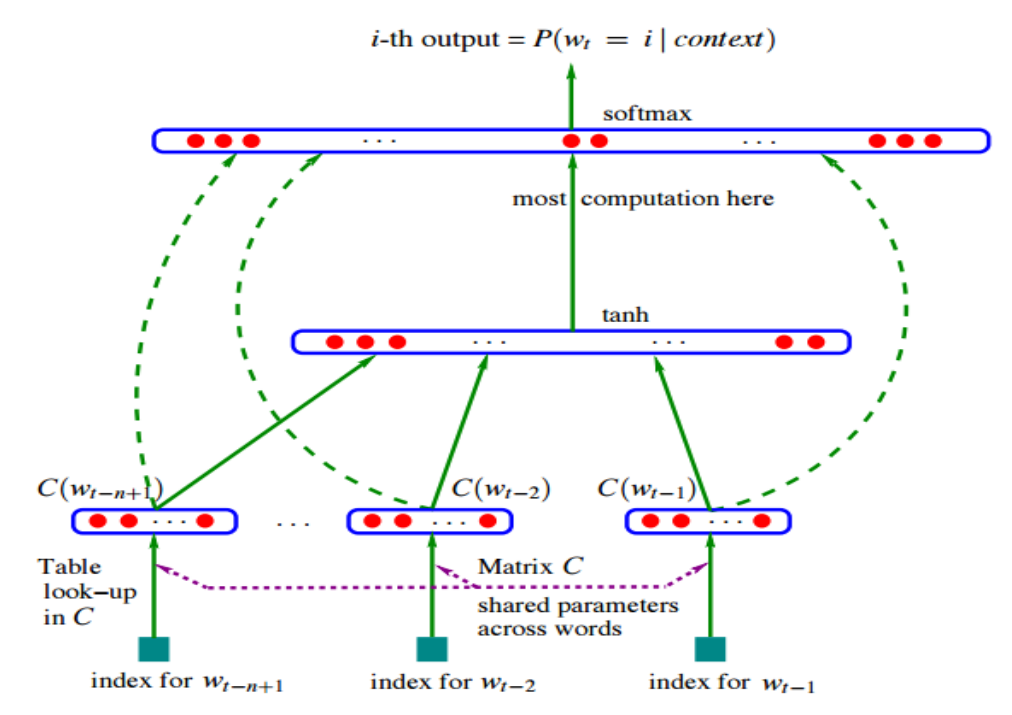## Word2Vec

### 简介

word2vec模型中比较重要的概念是词汇的上下文, 说白了就是一个词周围的词, 比如$w_t$的范围为1的上下文就是$w_{t-1}$$w_{t+1}$. 在word2vec中提出两个模型(假设上下文窗口为3)

• CBOW(Continuous Bag-of-Word): 以上下文词汇预测当前词: $w_{t-1}, w_{t+1}$ 去预测 $w_t$
• SkipGram: 以当前词预测其上下文词汇: $w_t$ 去预测$w_{t-1}, w_{t+1}$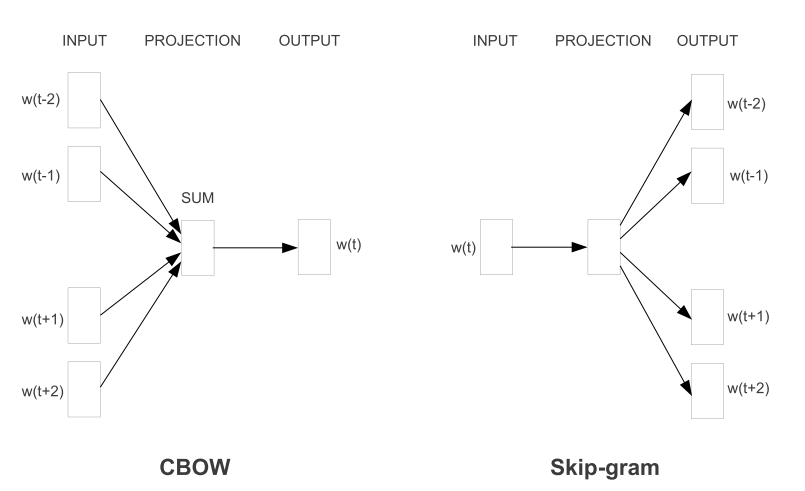### One-Word Model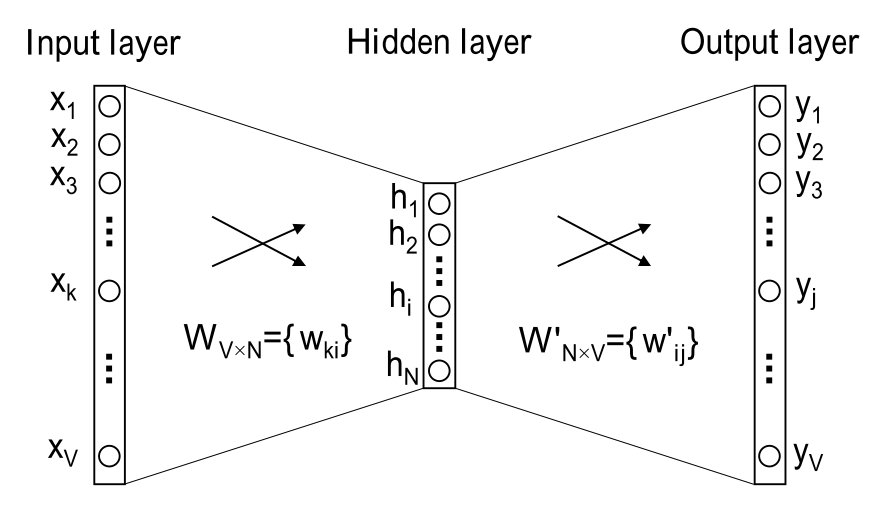• $V$: 词汇表长度; $N$: 隐层神经元个数, 同时也是词向量维度

• $W \in \mathcal{R}^{V\times N}$: 输入层到隐层的权重矩阵, 其实就是词向量矩阵,其中每一行代表一个词的词向量

• $W^{'} \in \mathcal{R}^{N\times V}$: 隐层到输出层的权重矩阵, 其中每一列也可以看作额外的一种词向量

$\mathbf{h} = W^T \cdot X = v_{w_I}^T$

$\mathbf{u} = W^{'T} \cdot h$

$P(w_j|w_I) = y_j = \frac{\exp(u_j)}{\sum\limits_{k\in V} \exp(u_k)} = \frac{\exp(v_{w_j}^{'T}\cdot v_{w_I})}{\sum\limits_{k\in V} \exp(v_{w_k}^{'T}\cdot v_{w_I})}$

\begin{align*} O &= \max P(w_O|w_I) \\ & = \max y_{j^{*}} := \max \log y_{j^{*}} \\ &= \max \log(\frac{\exp(u_{j^{*}})}{\sum \exp(u_k)}) = \max u_{j^{*}}-\log\sum_{k=1}^{V}\exp( u_k) \end{align*}

$E = -u_{j^{*}}+\log\sum_{k=1}^{V}\exp( u_k)$

$\frac{\partial E}{ \partial w{'}_{ij}} = \frac{\partial E}{\partial u_j} \frac{\partial u_j}{\partial w^{'}_{ij}} = (y_j-t_j) h_i$

$w^{'}_{ij} = {w^{'}_{ij}}^{(old)} -\eta (y_j - t_j) h_i$

$\mathbf{v}^{'}_{w_j} = {\mathbf{v}^{'}_{w_j}}^{(old)} - \eta(y_j-t_j)\mathbf{h}, \ j \in \{ 1,2,3,...,V\}$

$\frac{\partial E}{\partial h_i} = \sum_{j=1}^{V}\frac{\partial E}{\partial u_j} \frac{\partial u_j}{\partial h_i} = \sum_{j=1}^{V} (y_j-t_j)w^{'}_{ij} =W^{'}_{i} \cdot P$

$\frac{\partial E}{\partial \mathbf{h}} = W^{'} \cdot P$

$v_{w_I}^T = v_{w_I}^T - \eta W^{'} \cdot P$

### CBOW Model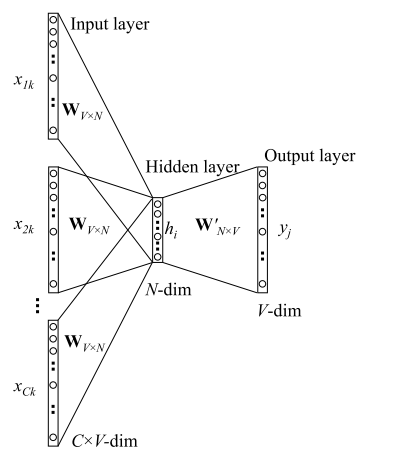\begin{align*} \mathbf{h} &= \frac{1}{C} W^T(x_{1} +x_2+...+x_{C}) \\ &= \frac{1}{C}(v_{w_1} + v_{w_2}+...+v_{w_C})^T\end{align*}

$\mathbf{v}^{'}_{w_j} = {\mathbf{v}^{'}_{w_j}}^{(old)} - \eta(y_j-t_j)\mathbf{h}, \ j \in \{ 1,2,3,...,V\}$

$\frac{\partial E}{\partial \mathbf{h}} = W^{'} \cdot P$

$v_{w_{I,c}}^T = v_{w_{I,c}}^T - \frac{1}{C}\ \eta W^{'} \cdot P,\ \ c=1,2,...,C$

### SkipGram Model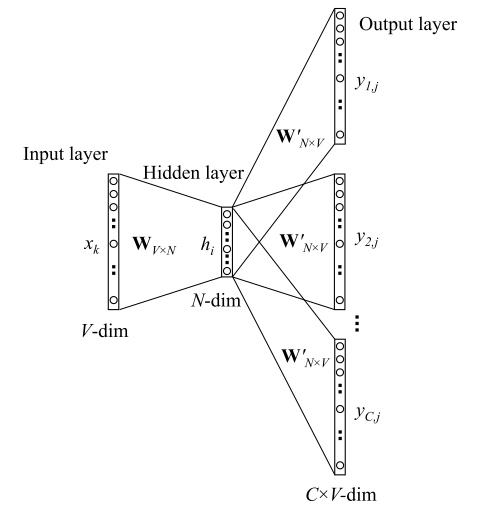$\mathbf{h} = W^T \cdot X = v_{w_I}^T$

$P(w_{c,j}|w_I) = y_{c,j} = \frac{\exp (u_{c,j})}{\sum_{k=1}^{V} \exp(u_{c,k})}$

\begin{align*}E &= -\log P(w_{1},w_{2},...,w_{C}| w_I) \\ &= - \log \Pi_{c=1}^{C}P(w_c|w_i) \\ &=-\log \Pi_{c=1}^{C} \frac{\exp (u_{c,j})}{\sum_{k=1}^{V} \exp(u_{c,k})} \\ &= -\sum_{c=1}^{C}u_{j_c^{*}} + C \cdot \log \sum_{k=1}^{V} \exp(u_{k}) \end{align*}

$\frac{\partial E}{\partial u_{c,j}} = y_{c,j} - t_{c,j}$

$\frac{\partial E}{\partial w^{'}_{ij}}= \sum_{c=1}^{C}\frac{\partial E}{\partial u_{c,j}} \frac{\partial u_{c,j}}{\partial w_{ij}^{'}} =\sum_{c=1}^{C}(y_{c,j}-t_{c,j})\mathbf{h_{i}} = Q_j \mathbf{h_i}$

$w_{i,j}^{'}={w_{ij}^{'}}^{(old)} - \eta Q_j\mathbf{h}_i$

$v_{w_j}^{'} = {v_{w_j}^{'}}^{(old)} - \eta Q_j \mathbf{h}, \ j = 1,2,3,...,V$

\begin{align*}\frac{\partial E}{\partial \mathbf{h}_i} &=\sum_{c=1}^{C}\sum_{j=1}^{V} \frac{\partial E}{\partial u_{c,j}} \frac{\partial u_{c,j}}{\partial \mathbf{h}_i} \\&=\sum_{c=1}^{C}\sum_{j=1}^{V}(y_{c,j}-t_{c,j}) w^{'}_{ij} \\ &= \sum_{j=1}^{V}Q_jw^{'}_{i,j}=W^{'}_{i} \cdot Q \end{align*}

$\frac{\partial E}{\partial \mathbf{h}} = W^{'} \cdot Q$

$v_{w_I}^T = v_{w_I}^T - \eta W^{'} \cdot Q$

## 优化

### Hierarchical SoftMax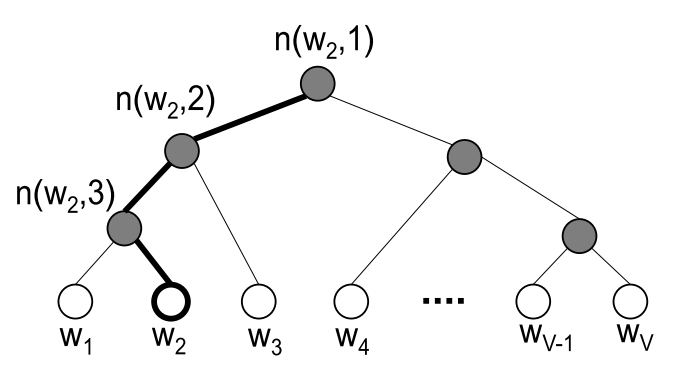\begin{align*}P(n, left ) &= \sigma({v^{'}_{w}} \cdot \mathbf{h}) \\ p(n, right) &=1-\sigma(v^{'}_w \cdot \mathbf{h}) = \sigma(-v_{w}^{'} \cdot h) \end{align*}

$P(w=w_O|w_I)= \Pi_{j=1}^{L(w)-1}P(\sigma(I(n(w,j+1==left) v^{'}_{w} \cdot \mathbf{h} ))$

\begin{align*}P(w_2=w_O) &= P(n(w_2,1), left) \cdot P(n(w_2,2), left) \cdot P(n(w_2, 3), right) \\ &= \sigma({v^{'}_{n(w_2,1)}}^T \mathbf{h}) \cdot \sigma({v^{'}_{n(w_2,2)}}^T \mathbf{h}) \cdot \sigma(-{v^{'}_{n(w_2,3)}}^T \mathbf{h}) \end{align*}

$\sum\limits_{j=1}^{V}P(w_j=w_O) =1$

$E = -\log P(w=w_O|w_I) = - \sum\limits_{j=1}^{L(w)-1}\log \sigma([I] {v^{'}_{j}}^{T} \mathbf{h})$

$\frac{\partial E}{\partial v^{'}_j} = \frac{\partial E}{\partial {v^{'}_{j}}^T \mathbf{h}} \frac{\partial {v^{'}_{j}}^T \mathbf{h}}{\partial {v^{'}_{j}}^T} = (\sigma( {v^{'}_{j}}^T \mathbf{h}) - t_j) \mathbf{h}$

${v^{'}_{j}}^{(new)} = {v^{'}_{j}}^{(old)} - \eta (\sigma( {v^{'}_{j}}^T \mathbf{h}) - t_j) \mathbf{h},\ j=1,2,3,...,L(w)-1$

\begin{align*} \frac{\partial E}{\partial \mathbf{h}} &= \sum\limits_{j=1}^{L(w)-1} \frac{\partial E}{\partial {v^{'}_{j}}^T \mathbf{h}} \frac{\partial {v^{'}_{j}}^T \mathbf{h}}{\partial \mathbf{h}} \\ &=\sum\limits_{j=1}^{L(w)-1}(\sigma( {v^{'}_{j}}^T \mathbf{h}) - t_j)\cdot v^{'}_{j}\end{align*}

### Negative Sampling

$weight(w) = \frac{coun(w)^{0.75}}{\sum _{u}count(w)^{0.75}}$

$E = -\log \sigma(v^{'}_{w_O}\mathbf{h}) - \sum\limits_{w_j \in \mathcal{W}_{neg}}\log \sigma(-v^{'}_{w_j}\mathbf{h})$

$\mathcal{W}_{neg}$是负例单词集合。 上述公式跟原本的负采样的有些许差别，具体的推导细节可以参考这篇Goldberg, Y. and Levy, O. (2014). word2vec explained: deriving mikolov et al.’s negative- sampling word-embedding method. 这里不再赘述了。 只需要记住，NS也是对softmax函数做的优化，除了word2vec，在其他地方涉及到softmax的均可以采用类似的思想来重写目标函数。

$\frac{\partial E}{\partial {v^{'}_{j}}^T \mathbf{h}} = \sigma( {v^{'}_{j}}^T \mathbf{h}) - t_j$

${v^{'}_{j}}^{(new)} = {v^{'}_{j}}^{(old)} - \eta (\sigma( {v^{'}_{j}}^T \mathbf{h}) - t_j) \mathbf{h},\ w_j \in \{w_O \} + \mathcal{W}_{neg}$

• HS实现比较复杂， 需要借助哈夫曼树，而且HS是专门优化softmax的方法
• NS相对简单，仅仅是简单采样，NS则是一种概率采样的方法，属于NCE(Noise Contrastive Estimation)的一种简单形式，代码实现也很简单。 训练得到的词向量质量也很高，相对常用一些。